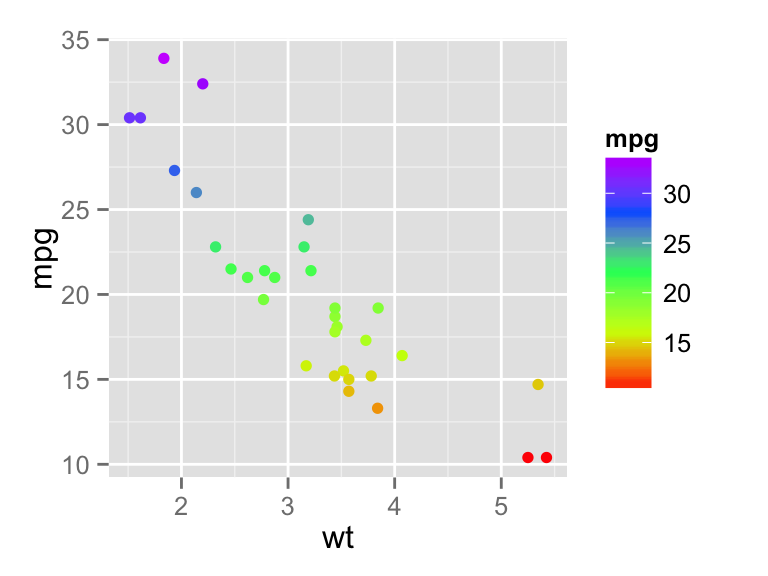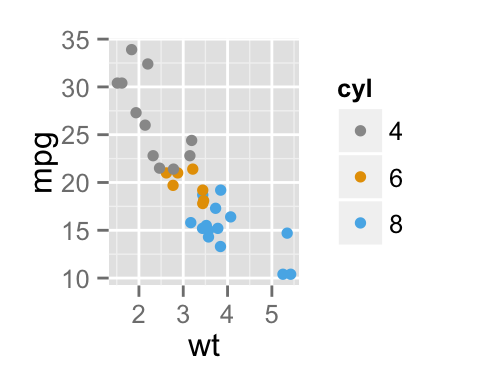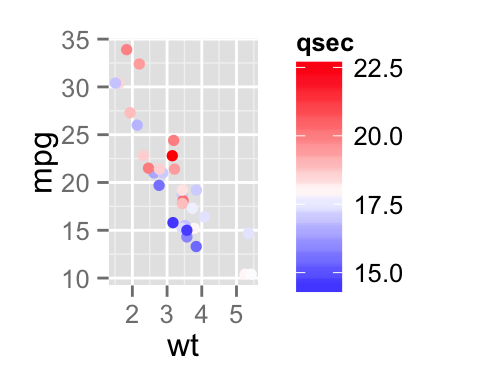# ggplot2 colors : How to change colors automatically and manually?

The goal of this article is to describe how to change the color of a graph generated using R software and ggplot2 package. A color can be specified either by name (e.g.: “red”) or by hexadecimal code (e.g. : “#FF1234”). The different color systems available in R are described at this link : colors in R.

In this R tutorial, you will learn how to :

• change colors by groups (automatically and manually)
• use RColorBrewer and Wes Anderson color palettes# Prepare the data

ToothGrowth and mtcars data sets are used in the examples below.

``````# Convert dose and cyl columns from numeric to factor variables
ToothGrowth\$dose <- as.factor(ToothGrowth\$dose)
mtcars\$cyl <- as.factor(mtcars\$cyl)
``````##    len supp dose
## 1  4.2   VC  0.5
## 2 11.5   VC  0.5
## 3  7.3   VC  0.5
## 4  5.8   VC  0.5
## 5  6.4   VC  0.5
## 6 10.0   VC  0.5``````
``head(mtcars)``
``````##                    mpg cyl disp  hp drat    wt  qsec vs am gear carb
## Mazda RX4         21.0   6  160 110 3.90 2.620 16.46  0  1    4    4
## Mazda RX4 Wag     21.0   6  160 110 3.90 2.875 17.02  0  1    4    4
## Datsun 710        22.8   4  108  93 3.85 2.320 18.61  1  1    4    1
## Hornet 4 Drive    21.4   6  258 110 3.08 3.215 19.44  1  0    3    1
## Hornet Sportabout 18.7   8  360 175 3.15 3.440 17.02  0  0    3    2
## Valiant           18.1   6  225 105 2.76 3.460 20.22  1  0    3    1``````

Make sure that the columns dose and cyl are converted as factor variables using the R script above.

# Simple plots

``````library(ggplot2)
# Box plot
ggplot(ToothGrowth, aes(x=dose, y=len)) +geom_boxplot()
# scatter plot
ggplot(mtcars, aes(x=wt, y=mpg)) + geom_point()``````# Use a single color

``````# box plot
ggplot(ToothGrowth, aes(x=dose, y=len)) +
geom_boxplot(fill='#A4A4A4', color="darkred")
# scatter plot
ggplot(mtcars, aes(x=wt, y=mpg)) +
geom_point(color='darkblue')``````# Change colors by groups

## Default colors

The following R code changes the color of the graph by the levels of dose :

``````# Box plot
bp<-ggplot(ToothGrowth, aes(x=dose, y=len, fill=dose)) +
geom_boxplot()
bp
# Scatter plot
sp<-ggplot(mtcars, aes(x=wt, y=mpg, color=cyl)) + geom_point()
sp``````The lightness (l) and the chroma (c, intensity of color) of the default (hue) colors can be modified using the functions scale_hue as follow :

``````# Box plot
bp + scale_fill_hue(l=40, c=35)
# Scatter plot
sp + scale_color_hue(l=40, c=35)``````Note that, the default values for l and c are : l = 65, c = 100.

## Change colors manually

A custom color palettes can be specified using the functions :

• scale_fill_manual() for box plot, bar plot, violin plot, etc
• scale_color_manual() for lines and points
``````# Box plot
bp + scale_fill_manual(values=c("#999999", "#E69F00", "#56B4E9"))
# Scatter plot
sp + scale_color_manual(values=c("#999999", "#E69F00", "#56B4E9"))``````Note that, the argument breaks can be used to control the appearance of the legend. This holds true also for the other scale_xx() functions.

``````# Box plot
bp + scale_fill_manual(breaks = c("2", "1", "0.5"),
values=c("red", "blue", "green"))
# Scatter plot
sp + scale_color_manual(breaks = c("8", "6", "4"),
values=c("red", "blue", "green"))``````The built-in color names and a color code chart are described here : color in R.

## Use RColorBrewer palettes

The color palettes available in the RColorBrewer package are described here : color in R.

``````# Box plot
bp + scale_fill_brewer(palette="Dark2")
# Scatter plot
sp + scale_color_brewer(palette="Dark2")``````The available color palettes in the RColorBrewer package are :## Use Wes Anderson color palettes

``````# Install
install.packages("wesanderson")
library(wesanderson)``````

The available color palettes are :``````library(wesanderson)
# Box plot
bp+scale_fill_manual(values=wes_palette(n=3, name="GrandBudapest"))
# Scatter plot
sp+scale_color_manual(values=wes_palette(n=3, name="GrandBudapest"))``````# Use gray colors

The functions to use are :

• scale_colour_grey() for points, lines, etc
• scale_fill_grey() for box plot, bar plot, violin plot, etc
``````# Box plot
bp + scale_fill_grey() + theme_classic()
# Scatter plot
sp + scale_color_grey() + theme_classic()``````Change the gray value at the low and the high ends of the palette :

``````# Box plot
bp + scale_fill_grey(start=0.8, end=0.2) + theme_classic()
# Scatter plot
sp + scale_color_grey(start=0.8, end=0.2) + theme_classic()``````Note that, the default value for the arguments start and end are : start = 0.2, end = 0.8

# Continuous colors

The graph can be colored according to the values of a continuous variable using the functions :

## Gradient colors for scatter plots

The graphs are colored using the qsec continuous variable :

``````# Color by qsec values
sp2<-ggplot(mtcars, aes(x=wt, y=mpg, color=qsec)) + geom_point()
sp2
# Change the low and high colors
# Sequential color scheme
# Diverging color scheme
mid<-mean(mtcars\$qsec)
high="red", space ="Lab" )``````## Gradient colors for histogram plots

``````set.seed(1234)
x <- rnorm(200)
# Histogram
hp<-qplot(x =x, fill=..count.., geom="histogram")
hp
# Sequential color schemeNote that, the functions scale_color_continuous() and scale_fill_continuous() can be used also to set gradient colors.

``````# Scatter plot
# Color points by the mpg variable
sp3<-ggplot(mtcars, aes(x=wt, y=mpg, color=mpg)) + geom_point()
sp3# Infos

This analysis has been performed using R software (ver. 3.1.2) and ggplot2 (ver. 1.0.0)